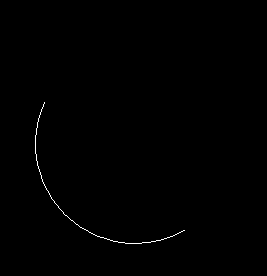# arc function in C

The header file graphics.h contains arc() function which draws an arc with center at (x, y) and given radius. start_angle is the starting point of angle and end_angle is the ending point of the angle. The value of the angle can vary from 0 to 360 degree.

Syntax :

```void arc(int x, int y, int start_angle,

where,
(x, y) is the center of the arc.
start_angle is the starting angle and
end_angle is the ending angle.
```

Examples :

```Input : x=250, y=250, start_angle = 155, end_angle = 300, radius = 100
Output :Input : x=250, y=250, start_angle = 0, end_angle = 300, radius = 100;
Output :```

## Recommended: Please try your approach on {IDE} first, before moving on to the solution.

Below is the implementation of arc() function :

 `// C implementation of arc function ` `#include ` ` `  `// driver code ` `int` `main() ` `{ ` `    ``// gm is Graphics mode which is ` `    ``// a computer display mode that ` `    ``// generates image using pixels. ` `    ``// DETECT is a macro defined in ` `    ``// "graphics.h" header file ` `    ``int` `gd = DETECT, gm; ` ` `  `    ``// location of the arc ` `    ``int` `x = 250; ` `    ``int` `y = 250; ` ` `  `    ``// starting angle and ending angle ` `    ``// of the arc ` `    ``int` `start_angle = 155; ` `    ``int` `end_angle = 300; ` ` `  `    ``// radius of the arc ` `    ``int` `radius = 100; ` ` `  `    ``// initgraph initializes the graphics system ` `    ``// by loading a graphics driver from disk ` `    ``initgraph(&gd, &gm, ``""``); ` ` `  `    ``// arc function ` `    ``arc(x, y, start_angle, end_angle, radius); ` ` `  `    ``getch(); ` ` `  `    ``// closegraph function closes the graphics ` `    ``// mode and deallocates all memory allocated ` `    ``// by graphics system ` `    ``closegraph(); ` ` `  `    ``return` `0; ` `} `

Output:

``````

Attention reader! Don’t stop learning now. Get hold of all the important DSA concepts with the DSA Self Paced Course at a student-friendly price and become industry ready.

My Personal Notes arrow_drop_upCheck out this Author's contributed articles.

If you like GeeksforGeeks and would like to contribute, you can also write an article using contribute.geeksforgeeks.org or mail your article to contribute@geeksforgeeks.org. See your article appearing on the GeeksforGeeks main page and help other Geeks.

Please Improve this article if you find anything incorrect by clicking on the "Improve Article" button below.

Improved By : Kirti_Mangal

Article Tags :
Practice Tags :

Be the First to upvote.

Please write to us at contribute@geeksforgeeks.org to report any issue with the above content.